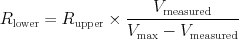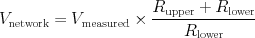# Chapter 8 Resistor Divider Networks

This module provides functions to calculate values from resistor divider networks.

## 8.1  Usage

To use the resistor divider network functions, use this:

  #include <resistor-network.h>


## 8.2  Functions

This function calculates the resistance of the lower resistor of a resistor divider network, given the maximum A/D counts v_ad_max, the measured A/D counts v_ad_counts for the divider network, and the known resistance r_upper in ohms of the upper resistor. It uses the equationto arrive at the result.

#### uint16_t divider_network_voltage(uint16_t v_ad_counts, uint16_t r_upper, uint16_t r_lower)function

This function calculates the voltage feeding into a resistor network given the measured A/D counts v_ad_counts, and the the known resistances in ohms of the upper resistor r_upper and the lower resistor r_lower. It uses the equationto arrive at the result.